Courses

# Chemistry- CY 2016 GATE Paper (Practice Test)

## 65 Questions MCQ Test GATE Past Year Papers for Practice (All Branches) | Chemistry- CY 2016 GATE Paper (Practice Test)

Description
This mock test of Chemistry- CY 2016 GATE Paper (Practice Test) for GATE helps you for every GATE entrance exam. This contains 65 Multiple Choice Questions for GATE Chemistry- CY 2016 GATE Paper (Practice Test) (mcq) to study with solutions a complete question bank. The solved questions answers in this Chemistry- CY 2016 GATE Paper (Practice Test) quiz give you a good mix of easy questions and tough questions. GATE students definitely take this Chemistry- CY 2016 GATE Paper (Practice Test) exercise for a better result in the exam. You can find other Chemistry- CY 2016 GATE Paper (Practice Test) extra questions, long questions & short questions for GATE on EduRev as well by searching above.
QUESTION: 1

Solution:
QUESTION: 2

Solution:
QUESTION: 3

### M has a son Q and a daughter R. He has no other children. E is the mother of P and daughter-inlaw of M. How is P related to M?

Solution:
QUESTION: 4

The number that least fits this set: (324, 441, 97 and 64) is ________.

Solution:
QUESTION: 5

It takes 10 s and 15 s, respectively, for two trains travelling at different constant speeds to
completely pass a telegraph post. The length of the first train is 120 m and that of the second train is
150 m. The magnitude of the difference in the speeds of the two trains (in m/s) is ____________.

Solution:
QUESTION: 6

Q. 6 – Q. 10 carry Two mark each.

Q.

The Velocity V of a vehicle along a straight line is measured in M/s and plotted as shown woth respect to time in seconds. At the end of the 7 seconds, how muc will the odometer reading increase by (in m) ?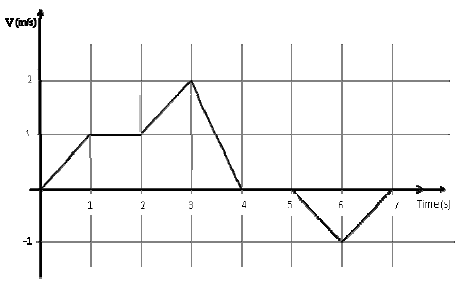Solution:
QUESTION: 7

The overwhelming number is people infected with rabies in India has been flagged by the world HEalth Organization as a source of concern. It is estimated that inoculating 70% of pets and stray dogs against rabies can lead to a significant reduction in the number of people infected with rabies.

Which of thel following can be logically inferred from the above sentence?

Solution:
QUESTION: 8

A flat is shared by four first year undergraduate students. They agreed to allow the oldest of them to enjoy some extra space in the flat. Manu is two months older than Sravan, who is three months younger than Trideep. Pavan is one month older than Sravan. Who should occupy the extra space in the flat?

Solution: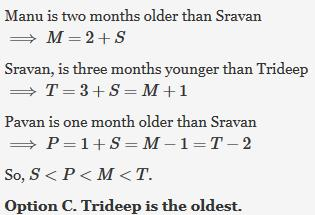QUESTION: 9

Find the area bounded by the lines 3x+2y =14, 2x-3y =5 in the first quadrant

Solution:
QUESTION: 10

A straight line is fit to a data set (ln x, y). This line intercepts the abscissa at ln x = 0.1 and has a
slope of −0.02. What is the value of y at x = 5 from the fit?

Solution:
QUESTION: 11

Q. 11 – Q. 35 carry one mark each.

Q.

[CpMoCl2]2 obeys the 18 electron rule. The correct structure of this compound is
(atomic number of Mo = 42)

Solution:
QUESTION: 12

During oxygen transport by hemerythrin, oxygen is bound as

Solution:
QUESTION: 13

Among the following, the most stable isotope to radioactive decay is

Solution:
QUESTION: 14

At pH 7.2 and 10 Torr oxygen partial pressure, the extent of O2 binding is

Solution:
QUESTION: 15

In the first row high-spin transition metal complexes [M(H2O)6]Cl2 with d5 and d7 metal ions, the
d-d transitions are

Solution:
QUESTION: 16

Among the given boranes and heteroboranes, the example which belongs to 'closo' type is

Solution:
QUESTION: 17

The reaction of P2O5 with HNO3 and HClO4, respectively, gives

Solution:
QUESTION: 18

When crystals of sodium chloride are heated in the presence of sodium vapor, they turn yellow.
This is due to the formation of

Solution:
*Answer can only contain numeric values
QUESTION: 19

One mole of an ideal gas is compressed from 5 L to 2 L at constant temperature. The change in
entropy, in J K-1, of the gas is ____________. (R = 8.314 J K-1 mol-1)

Solution:
QUESTION: 20

The linear momentum of a particle described by the wavefunction e-ikx is

Solution:
*Answer can only contain numeric values
QUESTION: 21

For an elementary bimolecular gas phase reaction, activation energy is 5.5 kJ mol-1. Enthalpy of
activation, in kJ mol-1, at 300 K is _____________. (R = 8.314 J K-1 mol-1)

Solution:
QUESTION: 22

The titration of a strong acid with a strong base is represented by the plot

Solution:
QUESTION: 23

Of the following inequalities, the criterion/criteria for spontaneity of a chemical reaction is/are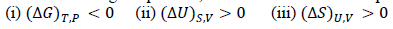Solution:
QUESTION: 24

A protein sample consists of an equimolar mixture of ribonuclease (molar mass = 13.7 kg mol?1),
hemoglobin (molar mass = 15.5 kg mol?1), and myoglobin (molar mass = 17.2 kg mol?1). The
statement that is true about the weight-average molar mass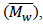the number-average molar mass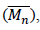and the polydispersity index (PDI) for this sample is

Solution:
QUESTION: 25

The band structure given below represents a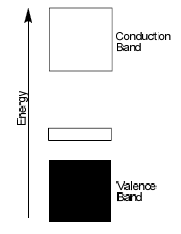Solution:
QUESTION: 26

The experimental ionization energies of hydrogen and helium atoms in their ground states are,
respectively, 13.6 eV and 24.6 eV. The ground state energy of helium atom, in eV, is

Solution:
QUESTION: 27

Ring flipping of the compound in the following conformation leads to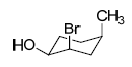Solution:
*Answer can only contain numeric values
QUESTION: 28

The total number of lines expected (due to spin-spin coupling of proton with fluorine and deuterium
nuclei) in the 1H NMR spectrum of the following compound is __________.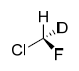Solution:
QUESTION: 29

The compound in 'R' configuration is

Solution:
QUESTION: 30

The most suitable reagent for performing the following transformation, is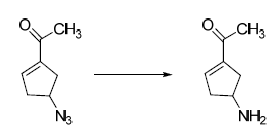Solution:
QUESTION: 31

The major product obtained in the following reaction, is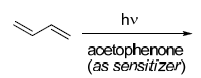Solution:
QUESTION: 32

The favourable transition state leading to the formation of the product in the following reaction, is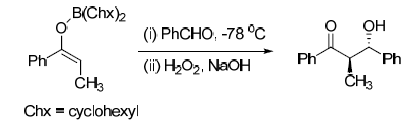Solution:
QUESTION: 33

The major product of the following reaction, is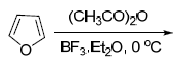Solution:
QUESTION: 34

The major product obtained in the following reaction, is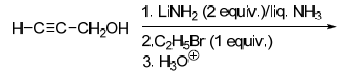Solution:
QUESTION: 35

The major product formed in the following reaction, is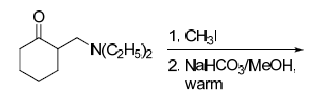Solution:
*Answer can only contain numeric values
QUESTION: 36

Q. 36 – Q. 65 carry two marks each

Q.

The Larmor frequency of 1H at 1 Tesla (T) is 42.57 MHz. If the magnetogyric ratios for 1H and 13C
are 26.75 × 107 rad T-1 s-1 and 6.72 × 107 rad T-1 s-1, respectively, the Larmor frequency of 13C, in
MHz, at 1 Tesla will be __________.

Solution:
*Answer can only contain numeric values
QUESTION: 37

At 1 bar and 298 K, for the process A(s) ? A(l), the ΔG is 200 J mol-1 and the ΔVm is −2 × 10-6
m3 mol-1. The minimum pressure, in bar, at which the process becomes spontaneous at 298 K is
_________. (1 bar = 105 Pa)

Solution:
*Answer can only contain numeric values
QUESTION: 38

The reaction, A ? B, is first order in both the directions. The forward and reverse rate constants are
4.2 × 10-4 s-1 and 1.04 × 10-3 s-1, respectively. The relaxation time for this reaction, in seconds, in a
temperature jump experiment is _____________.

Solution:
*Answer can only contain numeric values
QUESTION: 39

Adsorption of CO on charcoal at 273 K follows Langmuir isotherm. A plot of P (kPa) / V (cm3)
versus P (kPa) is linear with a slope of 0.01 and y-intercept of 0.5. The equilibrium constant, K
(kPa-1), for the adsorption is ___________.

Solution:
*Answer can only contain numeric values
QUESTION: 40

For the following reaction,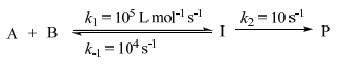if steady state approximation can be applied on [I], the observed rate constant of product formation,
in L mol-1 s-1, will be __________.

Solution:
QUESTION: 41

The correct set of infra-red spectral bands (in cm-1) for the νCO stretching mode of the given
carbonyl complex is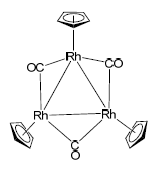Solution:
QUESTION: 42

The 19F NMR spectrum of ClF3 when measured at −60 ºC will be observed as a

Solution:
QUESTION: 43

Among the given platinum(II) complexes, the one that is thermally the most unstable is

Solution:
QUESTION: 44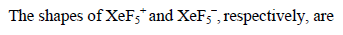Solution:
QUESTION: 45

Sodium salts of pseudohalogens X, Y, and Z form colourless solutions in water. Solution of X
decolorizes I?3 solution with brisk effervescence. Solution of Y gives an intense red colour on reaction with Fe3+ solution. Solution of Z gives an intense blue colour on reaction with a solution containing Fe3+ and Fe2+ ions. The pseudohalogens X, Y, and Z, respectively, are

Solution:
*Answer can only contain numeric values
QUESTION: 46

On reacting 1.55 g of a diol with an excess of methylmagnesium iodide, 1.12 L (corrected to STP)
of methane gas is liberated. The molecular mass (g mol-1) of the diol is ____________.

Solution:
QUESTION: 47

The structure of the compound having the following characteristic spectral data, is
IR: 1690 cm-1;
1H-NMR: 1.30 (3H, t, J = 7.2 Hz); 2.41 (2H, q, J = 7.2 Hz); 2.32 (3H, s); 7.44 (1H, t, J = 7.0 Hz);
7.57 (1H, dt, J = 7.0, 3.0 Hz); 7.77 (1H, t, J = 3.0 Hz); 7.90 (1H, dt, J = 7.0, 3.0 Hz);
EI Mass: m/z 119 (100%); 57 (80%).

Solution:
QUESTION: 48

The major products X and Y formed in the following synthetic scheme, are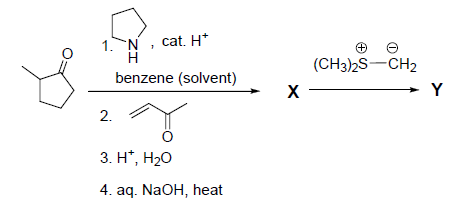Solution:
QUESTION: 49

The major products S and T formed in the following synthetic scheme, are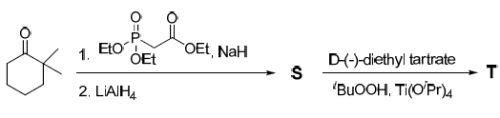Solution:
QUESTION: 50

Among the following, the transformation(s) that can be accomplished using umpolung concept
is(are)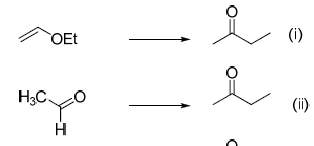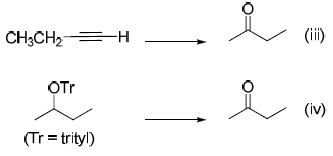Solution:
QUESTION: 51

A disaccharide does NOT give a positive test for Tollen's reagent. Upon acidic hydrolysis, it gives
an equimolar mixture of two different monosaccharides, both of which can be oxidized by bromine
water. This disaccharide is

Solution:
QUESTION: 52

The major products M and N in the following reaction sequence are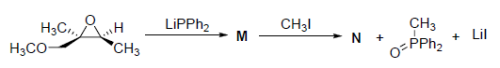Solution:
QUESTION: 53

The major products P and Q in the following reaction sequence, are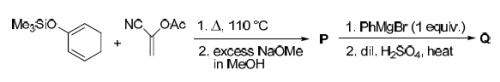Solution:
QUESTION: 54

The major product formed in the following reaction, is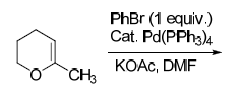Solution:
QUESTION: 55

The following synthetic transformation can be achieved using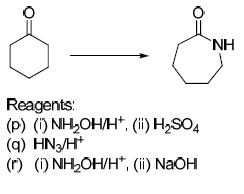Solution:
*Answer can only contain numeric values
QUESTION: 56

Consider a two-state system at thermal equilibrium with equal degeneracy where the excited state is
higher in energy than the ground state by 0.1 eV. The ratio of the population of the excited state to
that of the ground state, at a temperature for which kBT = 0.05 eV, is _________.

Solution:
QUESTION: 57

Of the vibrational modes given below, the IR active mode(s) is(are)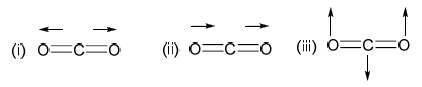Solution:
QUESTION: 58

A system is described by the following real wavefunction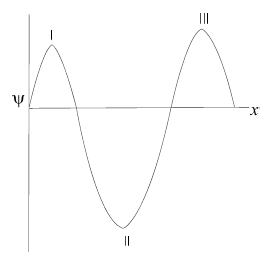The probability (P) of finding the particle in a region dx around points I, II, and III in the figure
obeys the trend

Solution:
QUESTION: 59

The temperature-composition (T-x) phase diagram of the two-component system made of X and Y
is given below. At a temperature of 288 K and starting at the point P, Y is added until the
composition reaches S. Which of the following statements is NOT TRUE?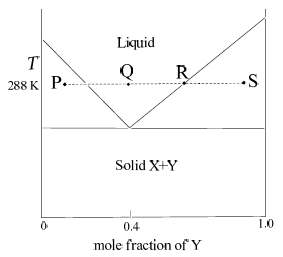Solution:
QUESTION: 60

For a system subjected to only P-V work, entropy is given by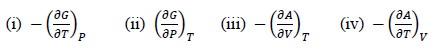Solution:
*Answer can only contain numeric values
QUESTION: 61

According to Irving-Williams series, the number of d electrons for the first row transition metal (M)
ion having the highest overall stability constant (log β) for [M(EDTA)]2- is ________.

Solution:
*Answer can only contain numeric values
QUESTION: 62

The magnitude of the difference in the crystal field stabilization energies, in Δo (ignoring pairing
energy), of [Fe(H2O)6]2+ and [Fe(CN)6]4- is _________.

Solution:
QUESTION: 63

The calculated and observed magnetic moments differ considerably for an aqua complex of a
lanthanide(III) ion as a result of low lying states of high J. The ion, among the following, is

Solution:
QUESTION: 64

In the electronic spectrum of [CrF6]3-, absorption bands observed at 670, 440, and 290 nm are,
respectively, due to the transitions

Solution:
QUESTION: 65

Amongst the following, the group that is bound to the metal ion in coenzyme B12 is

Solution: